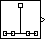# Discrete Impulse

Generate discrete impulse

• Library:
• DSP System Toolbox / Sources

•## Description

The Discrete Impulse block generates an impulse (the value `1`) at output sample `D+1`, where you specify `D` using the Delay parameter (`D``0`). All output samples preceding and following sample `D+1` are zero.

When `D` is a length-N vector, the block generates an M-by-N matrix output representing N distinct channels, where you specify frame size M using the Samples per frame parameter. The impulse for the `i`th channel appears at sample `D(i)+1`.

The Sample time parameter value, Ts, specifies the output signal sample period. The resulting frame period is MTs.

## Ports

### Output

expand all

Output signal containing a discrete impulse at output sample `D(i)+1`, where `D` is a scalar or vector specified by the Delay parameter. For more information, see Description.

Data Types: `single` | `double` | `int8` | `int16` | `int32` | `int64` | `uint8` | `uint16` | `uint32` | `uint64` | `Boolean` | `fixed point`

## Parameters

expand all

### Main

The number of zero-valued output samples, `D`, preceding the impulse, specified as a scalar or vector of integer values, greater than or equal to zero. A length-N vector specifies an N-channel output.

The sample period, Ts, of the output signal specified as a positive finite scalar. The output frame period is MTs.

The number of samples, M, in each output frame, specified as a positive integer scalar.

### Data Types

Specify the output data type for this block. You can select one of the following:

• A rule that inherits a data type, for example, ```Inherit: Inherit via back propagation```. When you select this option, the output data type and scaling matches that of the next downstream block.

• A built-in data type, such as `double`

• An expression that evaluates to a valid data type, for example, `fixdt(1,16)`

For help setting data type parameters, display the Data Type Assistant by clicking the button.

## Block Characteristics

 Data Types `Boolean` | `double` | `fixed point` | `integer` | `single` Direct Feedthrough `no` Multidimensional Signals `no` Variable-Size Signals `no` Zero-Crossing Detection `no`

## Extended Capabilities

### Topics

Introduced before R2006a

Watch now